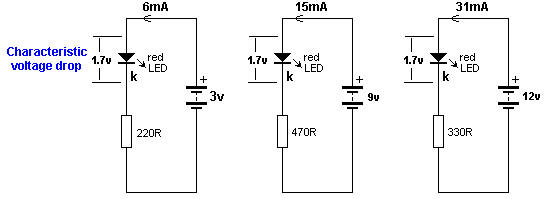Characteristic voltage drop
Electronic

When a LED is connected around the correct way in a circuit it develops a voltage across it called the
CHARACTERISTIC VOLTAGE DROP.

A LED must be supplied with a voltage that is higher than its "CHARACTERISTIC VOLTAGE" via a resistor - called a VOLTAGE DROPPING RESISTOR or CURRENT LIMITING RESISTOR - so the LED will operate correctly and provide at least 10,000 to 50,000 hours of illumination.

A LED works like this:

A LED and resistor are placed in series and connected to a voltage.

As the voltage rises from 0v, nothing happens until the voltage reaches about 1.7v.

At this voltage a red LED just starts to glow.

As the voltage increases, the voltage across the LED remains at 1.7v but the current through the LED increases and it gets brighter.

We now turn our attention to the current though the LED.

As the current increases to 5mA, 10mA, 15mA, 20mA the brightness will increase and at 25mA, it will be a maximum.

Increasing the supply voltage will simply change the color of the LED slightly but the crystal inside the LED will start to overheat and this will reduce the life considerably.

This is just a simple example as each LED has a different CHARACTERISTIC VOLTAGE DROP and a different maximum current.

In the diagram below we see a LED on a 3v supply, 9v supply and 12v supply.

The current-limiting resistors are different and the first circuit takes 6mA, the second takes 15mA and the third takes 31mA.

But the voltage across the red LED is the same in all cases.

This is because the LED creates the CHARACTERISTIC VOLTAGE DROP and this does not change.It does not matter if the resistor is connected above or below the LED.

The circuits are the SAME in operation:Recherche personnalisée